# Span-wise Fluctuating Mhd Convective Heat and Mass Transfer Flow Through Porous Medium in a Vertical Channel with Thermal Radiation and Chemical Reaction

Span-wise Fluctuating Mhd Convective Heat and Mass Transfer Flow Through Porous Medium in a Vertical Channel with Thermal Radiation and Chemical Reaction

Alphonsa Mathew K. D. Singh

Department of mathematics, St. Thomas College, Trissur, Kerala, India;

Professor of Applied Mathematics, Wexlow, Lower Kaithu, Shimla-171003, India.t

Corresponding Author Email:
alphonsastc@gmail.com; kdsinghshimla@gmail.com
Page:
135-142
|
DOI:
https://doi.org/10.18280/ijht.330222
N/A
|
Accepted:
N/A
|
Published:
30 June 2015
| Citation

OPEN ACCESS

Abstract:

An exact solution of span-wise fluctuating magnetohydrodynamic (MHD) convective flow problem of a viscous, incompressible and electrically conducting fluid through a porous medium filled in an infinite vertical channel is obtained. The channel walls at $y^{*}=-\frac{d}{2}$ and at $y^{*}=+\frac{d}{2}$ are subjected to span-wise cosinusoidally varying species concentration and temperature. A magnetic field of uniform strength is applied perpendicular to the planes of the channel plates. The magnetic Reynolds number is assumed very small so that the induced magnetic field is neglected. The temperature difference between the plates is high enough to induce the heat due to radiation. The Rosseland approximation is used to describe the radiation heat flux for the fluid as optically-thick gray gas, absorbing/emitting but non-scattering medium. The partial differential equations governing the flow are solved exactly under the prescribed boundary conditions for the velocity, temperature and species concentration fields. The velocity, temperature, concentration and the skin-friction, Nusselt number, Sherwood number in terms of their amplitudes and phase angles have been shown graphically to observe the effects of different flow parameters. The final results are then discussed in detail in the last section of the paper with the help of figures.

Keywords:

Magnetohydromagnetic (MHD), convective, span-wise fluctuating, viscoelastic, porous medium, radiation.

1. Introduction

The phenomenon of heat and mass transfer has attracted the attention of large number of scholars due to its applications in Science and Technology. Such phenomena are observed in buoyancy induced motions in the atmosphere, in bodies of water, quasi-solid bodies such as earth and so on.  Process involving coupled heat and mass transfer occur frequently in nature. It occurs not only due to temperature difference, but also due to concentration difference or the combination of these two. The study of heat and mass transfer due to chemical reaction is also very importance because of its occurrence in most of the branches of science and technology. The processes involving mass transfer effects are important in chemical processing equipments which are designed to draw high value products from cheaper raw materials with the involvement of chemical reaction. In many industrial processes, the species undergo some kind of chemical reaction with the ambient fluid which may affect the flow behavior and the production quality of final products. Kandasamy et al.  discussed the effects of chemical reaction and magnetic field on heat and mass transfer over a vertical stretching surface. Muthucumaraswamy and Janakiraman  analyzed the effects of mass transfer over a vertical oscillating plate with chemical reaction. The effect of mass transfer on flow past an impulsively started infinite vertical plate with constant heat flux and chemical reaction were studied by Das et al. . Mansour et al.  studied the effects of chemical reaction and viscous dissipation on MHD natural convection flows saturated in porous media.

Radiative heat and mass transfer play an important role in manufacturing industries for the design of reliable equipment. Radiation effect on mixed convection along an isothermal vertical plate was studied by Hossain and Takhar . Cookey et al.  have investigated unsteady two-dimensional flow of a radiating and chemically reacting MHD fluid with time dependent suction. Kesavaiah et al.  investigated effects of the chemical reaction and radiation absorption on an unsteady MHD convective heat and mass transfer flow past a semi-infinite vertical permeable moving plate embedded in a porous medium with heat source and suction.

There are many material processing industrial operations where due to high temperatures all the three modes of heat transportation such as conduction, convection and radiation accompany together. Majority of studies are confined to the situations where the wall temperature is either constant or varies with time only. But there are many industrial and engineering processes where the temperature may vary in a rather complex manner. For example the temperature may vary in certain direction in addition to time variation. A few studies have been conducted by considering spanwise cosinusoidal temperatures of the surfaces. Singh  analyzed an unsteady free convection flow past a hot vertical porous plate with variable temperature. Singh and Khem Chand  discussed an unsteady free convective MHD flow past a vertical porous plate with variable temperature. Sumathi et al. also attempted heat and mass transfer in an unsteady three dimensional mixed convection flow past an infinite vertical porous plate with cosinusoidally fluctuating temperature. Kumar and Singh  studied an unsteady MHD flow of radiating and reacting fluid past a vertical porous plate with cosinusoidally fluctuating temperature. In all these studies the problem of such a variation of the temperature is treated for the flow past infinite plate only.

An attempt has been made in this paper to study the MHD mixed convection heat and mass transfer flow through porous medium in a vertical channel with chemical reaction and radiation. It is also assumed that the conducting fluid is optically-thick gray gas, absorbing/ emitting radiation and non-scattering. An exact solution of the mathematical problem is obtained and the final results for the velocity, temperature, shear stress and heat transfer coefficient in terms of their amplitudes and phase angles are discussed in the last section of the paper.

2. Mathematical Analysis

An unsteady MHD convective flow of a viscous, incompressible and electrically conducting fluid through a porous medium in a vertical channel is considered. The channel plates are distance 'd' apart  are subjected to span-wise cosinusoidally varying species concentration and temperature. The $x^{*}$- axis is oriented vertically upwards along the centreline of the channel. The $y^{*}$-axis taken perpendicular to the planes of the plates and a transverse magnetic field of uniform strength $\vec{B}=\left(0, B_{0}, 0\right)$ is applied along this axis. The non-uniform species concentration and temperature of the plate at $y^{*}=-\frac{d}{2}$ and at $y^{*}=+\frac{d}{2}$ respectively are assumed to be varying span-wise cosinusoidally in space and time both as

$c^{*}\left(y^{*}, z^{*}, t^{*}\right)=C_{1}+\left(C_{2}-C_{1}\right) \cos \left(\frac{\pi z^{*}}{d}-\omega^{*} t^{*}\right)$    (1)

$T^{*}\left(y^{*}, z^{*}, t^{*}\right)=T_{1}+\left(T_{2}-T_{1}\right) \cos \left(\frac{\pi z^{*}}{d}-\omega^{*} t^{*}\right)$    (2)

The physical configuration of the problem is shown in Figure 1a, 1b & 1c.

## 1a.pngFigure 1a. Hot vertical channel.

## 1b.pngFigure 1b. Span-wise cosinusoidal species concentration.

## 1c.png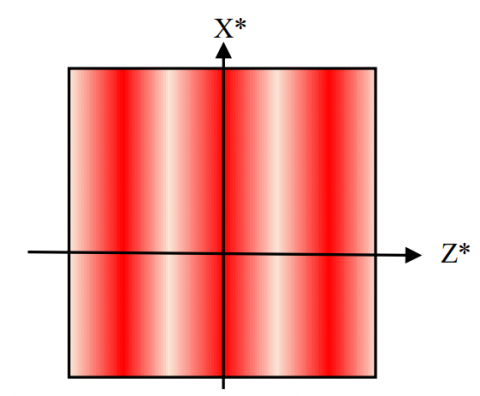Figure 1c. Span-wise cosinusoidal plate temperature.

Since the plates of the channel are of infinite extent in the x*-direction, therefore, all the physical quantities except the pressure are independent of x*. All fluid properties are assumed to be constant except that the influence of density variation with temperature is considered only in the body force term. The equation of continuity $\nabla \cdot \vec{V}=0$, where $\vec{V}=\left(u^{*}, v^{*}, 0\right)$, for impermeable channel plates integrates to v*= 0.

Under the usual Boussinsq’s approximation the magnetohydrodynamic (MHD) mixed convection flow in the vertical channel with chemical reaction and thermal radiation is governed by the following partial differential equations:

$\frac{\partial u^{*}}{\partial t^{*}}=-\frac{1}{\rho} \frac{\partial p^{*}}{\partial x^{*}}+\vartheta\left(\frac{\partial^{2} u^{*}}{\partial y^{* 2}}+\frac{\partial^{2} u^{*}}{\partial z^{* 2}}\right)-\frac{\sigma \bar{B}_{0}^{2}}{\rho} u^{*}-\frac{\theta}{k^{*}} u^{*}+$

$g \beta\left(T^{*}-T_{1}\right)+g \beta^{*}\left(C^{*}-C_{1}\right)$      (3)

$\rho c_{p} \frac{\partial \tau^{*}}{\partial t^{*}}=k\left(\frac{\partial^{2} \tau^{*}}{\partial y^{* 2}}+\frac{\partial^{2} \tau^{*}}{\partial z^{* 2}}\right)-\frac{\partial q^{*}}{\partial y^{*}}$         (4)

$\frac{\partial c^{*}}{\partial t^{*}}=D\left(\frac{\partial^{2} C^{*}}{\partial y^{* 2}}+\frac{\partial^{2} c^{*}}{\partial z^{* 2}}\right)-R^{*}\left(C^{*}-C_{1}\right)$     (5)

where ρ is the density, $\theta$ is the kinematic viscosity, β is the coefficient of volume expansion, β* is the volumetric coefficient of expansion with concentration, p* is the pressure, g is the acceleration due to gravity, k is the thermal conductivity, cp is the specific heat at constant pressure, D is the molecular diffusivity and R* is the chemical reaction.

Following Siegel and Howell , the last term in equation (4) stands for the heat flux due to radiation and for an optically thick gray gas is expressed by using Rosseland approximation as

$q^{*}=-\frac{4 \sigma^{*}}{3 k^{*}} \frac{\partial \tau^{* 4}}{\partial y^{*}}$      (6)

where$\sigma^{*}$ is Stefan- Boltzmann constant and k* is the mean absorption coefficient. We assume that the temperature differences within the flow are sufficiently small such that T*4 may be expanding in Taylor series about T1. Neglecting higher order terms and retaining first term only, we obtain

$T^{* 4} \cong 4 T_{1}^{3} T^{*}-3 T_{1}^{4}$       (7)

Substituting (7) into (6) and simplifying, we obtain

$\frac{\partial q^{*}}{\partial y^{*}}=-\frac{16 \sigma^{*} \tau_{1}^{3}}{3 k^{*}} \frac{\partial^{2} \tau^{*}}{\partial y^{* 2}}$     (8)

The substitution of equation (8) into the energy equation (4) for the heat due to radiation, we get

$\rho c_{p} \frac{\partial \tau^{*}}{\partial t^{*}}=k\left(\frac{\partial^{2} \tau^{*}}{\partial y^{* 2}}+\frac{\partial^{2} \tau^{*}}{\partial z^{* 2}}\right)+\frac{16 \sigma^{*} T_{1}^{3}}{3 k^{*}} \frac{\partial^{2} \tau^{*}}{\partial y^{* 2}}$     (9)

The boundary conditions for the problem are

$y^{*}=-\frac{d}{2}: u^{*}=0, T^{*}=T_{1}, C^{*}=C_{1}+ \left(C_{2}-C_{1}\right) \cos \left(\frac{\pi z^{*}}{d}-\omega^{*} t^{*}\right)$     (10)

$y^{*}=+\frac{d}{2}: u^{*}=0, T^{*}=T_{1}+\left(T_{2}-T_{1}\right) \cos \left(\frac{\pi z^{*}}{d}-\omega^{*} t^{*}\right), C^{*}= C_{1}$    (11)

Introducing the following non-dimensional quantities

$x, y, z=\frac{x^{*}, y^{*}, z^{*}}{d}, t=\omega^{*} t^{*}, \omega=\frac{\omega^{*} d^{2}}{\theta}, u=\frac{u^{*}}{U}, \theta=\frac{\tau^{*}-\tau_{1}}{\tau_{2}-\tau_{1}}$

$C=\frac{c^{*}-c_{1}}{c_{2}-c_{1}}, p=\frac{d p^{*}}{\rho \theta U^{\prime}}$      (12)

into equations (3) to (5) we get

$\omega \frac{\partial u}{\partial t}=-\frac{\partial p}{\partial x}+\left(\frac{\partial^{2} u}{\partial y^{2}}+\frac{\partial^{2} u}{\partial z^{2}}\right)-\left(M^{2}+K^{-1}\right) u+G r \theta+G m C$    (13)

$\omega P r \frac{\partial \theta}{\partial t}=\left(1+\frac{4}{3 N}\right) \frac{\partial^{2} \theta}{\partial y^{2}}+\frac{\partial^{2} \theta}{\partial z^{2}}$     (14)

$\omega S c \frac{\partial c}{\partial t}=\frac{\partial^{2} c}{\partial y^{2}}+\frac{\partial^{2} c}{\partial z^{2}}-S c R C$      (15)

where ‘*’ represents the dimensional physical quantities,

$G r=\frac{g \beta d^{2}\left(\tau_{2}-\tau_{1}\right)}{\theta U}$ is the Grashof number,

$G m=\frac{g \beta^{*} d^{2}\left(c_{2}-c_{1}\right)}{\theta v}$ is the modified Grashof number,

$M=B_{0} d \sqrt{\frac{\sigma}{\mu}}$ is the Hartmann number,

$K=\frac{K^{*}}{d^{2}}$ is the permeability of the porous medium,

$P r=\frac{\mu c_{p}}{k}$ is the Prandtl number,

$N=\frac{k k^{*}}{4 \sigma^{*} \tau_{1}^{3}}$ is the radiation parameter,

$S c=\frac{\theta}{D}$ is the Schmidt number,

$R=\frac{K_{r}^{*} d^{2}}{\theta}$ is the chemical reaction parameter.

The boundary conditions in the dimensionless form become

$y=-\frac{1}{2}: u=0, \theta=0, C=\cos (\pi z-t)$   (16)

$y=\frac{1}{2}: u=0, \theta=\cos (\pi z-t)$    (17)

In order to obtain the solution of this problem when the fluid is acted upon by an unsteady span-wise drop in pressure, we assume the solution in complex variable notations as

$u(y, z, t)=u_{0}(y) e^{i(\pi z-t)}, \theta(y, z, t)=\theta_{0}(y) e^{i(\pi z-t)}$

$C(y, z, t)=C_{0}(y) e^{i(\pi z-t)},-\frac{\partial p}{\partial x}=A e^{i(\pi z-t)}$   (18)

where A is a constant. The real part of the solution will have physical significance.

The boundary conditions (16) and (17) can also be written in complex notations as

$y=-\frac{1}{2}: u=0, \theta=0, C=e^{i(\pi z-t)}$         (19)

$y=\frac{1}{2}: u=0, \theta=e^{i(\pi z-t)}, C=0$     (20)

Substituting expressions (18) into equations (13) to (15), we obtain following equations

$u_{0}^{\prime \prime}-\left(\pi^{2}+M^{2}+K^{-1}-i \omega\right) u_{0}=-A-G r \theta_{0}-G m C_{0}$  (21)

$\left(1+\frac{4}{3 N}\right) \theta_{0}^{\prime \prime}-\left(\pi^{2}-i \omega P r\right) \theta_{0}=0$        (22)

$C_{0}^{\pi}-\left(\pi^{2}+S c R-i \omega S c\right) C_{0}$      (23)

where the primes in these ordinary differential equations denote differentiation with respect to y. The boundary conditions (19) and (20) reduce to

$y=-\frac{1}{2}: u_{0}=0, \theta_{0}=0, C_{0}=1$      (24)

$y=\frac{1}{2}: u_{0}=0, \quad \theta_{0}=1, C_{0}=0$      (25)

The solution of equation (21) for the velocity field under the boundary conditions (24) and (25) is obtained as

$u(y, z, t)=\frac{A}{m^{2}}\left[1-\frac{\cosh m y}{\cosh ^{m} / 2}\right]+\frac{G r}{n^{2}-m^{2}}\left[\frac{\sinh m\left(\frac{1}{2}+y\right)}{\sinh m}-\right.$

$\left.\frac{\sinh n\left(\frac{1}{2}+y\right)}{\sinh n}\right]+\frac{G m}{l^{2}-m^{2}}\left[\frac{\sinh m\left(\frac{1}{2}-y\right)}{\sinh m}-\frac{\sinh l\left(\frac{1}{2}-y\right)}{\sinh l}\right] e^{i(\pi z-t)}$   (26)

where $l=\sqrt{\pi^{2}+S c R-i \omega S c}$,$n=\sqrt{\frac{\pi^{2}-i \omega P r}{1+\frac{4}{3 N}}}$ , $m=\sqrt{\pi^{2}+M^{2}+K^{-1}-i \omega}$.

Similarly, the solutions of equations (22) and (23) for temperature and species concentration under the boundary conditions (24) and (25) are obtained as

$\theta(y, z, t)=\frac{\sinh n\left(\frac{1}{2}+y\right)}{\sinh n} e^{i(\pi z-t)}$      (27)

$C(y, z, t)=\frac{\sinh l\left(\frac{1}{2}-y\right)}{\sinh l} e^{i(\pi z-t)}$    (28)

From the velocity field obtained in equation (26) we can get the skin-friction $\tau_{L}$ at the left plate (y = -0.5) in terms of its amplitude $|F|$ and phase angle φ as

$\tau_{L}=|\mathrm{F}| \cos (\pi \mathrm{z}-\mathrm{t}+\varphi)$ , with

$F=F_{r}+i F_{i}=\frac{A}{m} \tanh m / 2+\frac{G r}{n^{2}-m^{2}}\left[\frac{m}{\sinh m}-\frac{n}{\sinh n}\right]+$

$\frac{G m}{l^{2}-m^{2}}[m \operatorname{coth} m-l \operatorname{coth} l]$     (29)

The amplitude and the phase angle of skin friction are

$|\mathrm{F}|=\sqrt{\mathrm{F}_{\mathrm{r}}^{2}+\mathrm{F}_{\mathrm{i}}^{2}}$ and $\varphi=\tan ^{-1} \frac{\mathrm{F}_{\mathrm{i}}}{\mathrm{F}_{\mathrm{r}}}$   (30)

Similarity, we can get the Nusselt number, Nu, and the Sherwood number Sh in terms of their respective amplitudes $|H|$ and $|C|$ the phase angles $\psi$ and $\xi$ from solutions (27) and (28) as

$N u=|H| \cos (\pi z-t+\psi)$   (31)

$S h=|C| \cos (\pi z-t+\zeta)$      (32)

with

$H=H_{r}+i H_{i}=\frac{n}{\sinh n}$    (33)

and

$C=C_{r}+i C_{i}=-l$ coth $l$     (34)

where $|H|$, $|C|$ are the amplitudes and ψ, ζ  are the phase angle of Nu, Sh  respectively are given as

$|H|=\sqrt{H_{r}^{2}+H_{i}^{2}}, \quad \psi=\tan ^{-1} \frac{H_{i}}{H_{r}}$    (35)

and

$|C|=\sqrt{C_{r}^{2}+C_{i}^{2}} \quad \quad \zeta=\tan ^{-1} \frac{c_{i}}{c_{r}}$  (36)

3. Discussion

To have a detailed insight of the problem the exact solutions obtained above are evaluated numerically for different sets of parameters involved. The influence of each of these parameters on the physical quantities like the velocity, temperature, species concentration, amplitudes and phase angles of skin-friction, Nusselt number and Sherwood number are shown graphically.

The effects of different parameters on the velocity field u(y, z, t) are shown in Figure 2. Different curves in this figure represent the sets of various values of the parameters listed in Table 1. This figure clearly shows that the velocity is maximum in the middle of the channel which leads to parabolic velocity profiles in the channel as expected. To assess the influence of each parameter on the velocity every curve is compared with dotted curve I (---). This figure clearly shows that curves II, III, and V lie above the dashed curve I (---) which means that the velocity increases with the increase of Grashof number Gr, modified Grashof number Gm and permeability of the porous medium K. The increase of velocity u(y, z, t) with the increase of Gr and Gm physically means that the enhancement of the buoyancy force due to temperature and concentration variations lead to the increase of the velocity. The increase of velocity with the increase of permeability of the porous medium indicates that the resistance posed by the porous medium reduces as the permeability of the medium increases because of which the velocity increases.

## 1c.pngFigure 2. Velocity profiles for A=2, z=0.5 and t=π/2.

The effects of other parameters like Hartmann number M, Prandtl number Pr, radiation parameter N, Schmdt number Sc, reaction parameter R and frequency of oscillations ω are represented by curves IV, VI, VII, VIII, IX and X respectively. These curves lie below dotted curve II (---). This means that the flow velocity decreases with the increase of these parameters. Lorentz force which is introduced due to the application of the transverse magnetic field retards the velocity. This force gives a dragging effect on the flow. The two values of the Prandtl number Pr=0.7 and Pr=7 are chosen to represent most common fluids air and water respectively. It is evident that the velocity is less in water than in air. Since the Prandtl number gives the relative importance of viscous dissipation to the thermal dissipation so for larger Prandtl number viscous dissipation is predominant and due to this velocity decreases. Similarly the two values of Schmidt number Sc=0.22 and 0.94 chosen represent Hydrogen and Carbon dioxide respectively. The velocity decreases with increasing Sc. The increase of radiation N and the frequency ω leads to a decrease in velocity.

## t1.png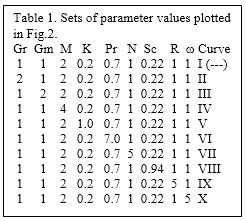Figure 3 show the variation of temperature with Prandtl number Pr,radiation parameter N and frequency of oscillations $\omega$.The comparison of different curves with the dotted curve  (---) reveals that the temperature decreases with the increase of either of these parameters.

## 3.pngFigure 3. Temperature profiles for z=0.5 and t=π/2.

Similarly, the comparison of different curves with the dotted curve in figure 4 depicts that the species concentration also decreases with the increase of either of the Schmidt number Sc, reaction parameter R and the frequency of oscillations ω.

## 4.png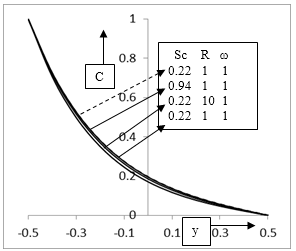Figure 4. Species concentration for z=0.5 and t=π/2.

The skin-friction $\tau_{2}$ in terms of its amplitude $|F|$ and phase angle $\varphi$ has been shown in Figures. 5 and 6 respectively for the sets of values listed in Table $2 .$ In Figure.5 the comparison of the curves II, $\mathrm{V}, \mathrm{VIII}$ and $\mathrm{IX}$ with dotted curve I $(---)$ indicate that the amplitude increases with the increase of Grashof number Gr, permeability of the porous medium $\mathrm{K}$ Schmidt number Sc and chemical reaction R. Similarly, the comparison of the curves III, IV, VI, and VII, with dotted curve I (- - - ) reveals that the skin-friction amplitude decreases with the increase modified Grashof number Gm, Hartmann number $\mathrm{M}$, Prandtl number Pr and radiation parameter $\mathrm{N}$. It is obvious that $|F|$ goes on decreasing with increasing frequency of oscillations $\omega .$ Figure 6 clearly shows that there is always a phase lead because the values of $\varphi$ remain positive throughout. It is found by comparing curves II, III, V and IX with dotted curve I (- - -) that the phase lead increases with the increase Grashof number Gr, modified Grashof number Gm, permeability of the porous medium K and reaction parameter $\mathrm{R}$. Also the comparison of curves $\mathrm{IV}, \mathrm{VI}$ VII and VIII with dotted curve I (- - - ) indicate that the phase lead decreases with the increase of Hartmann number $\mathrm{M}$ Prandtl number Pr, radiation $\mathrm{N},$ and Schmidt number Sc. Phase lead goes on increasing with increasing frequency of oscillations $\omega$

## 5.pngFigure 5. Amplitude of skin-friction.

## t2.png## 6.png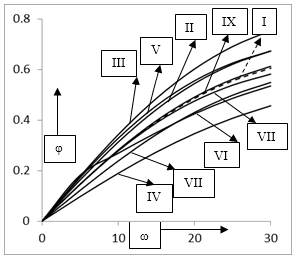Figure 6. Phase of skin friction.

The amplitude $|H|$ and the phase angle of the Nusselt number Nu are presented in Figures 7 and 8 respectively and the curves are compared with dotted (---) curve. Figure 7 reveals that $|H|$ decreases sharply with the increase of Prandtl number or radiation parameter as frequency ω increases. The amplitude remains almost constant with increasing frequency of oscillations ω. The phase angle ψ of rate of heat transfer shown in figure 8 oscillates between phase lag and phase lead as ω increases. The wave length due to the increase of Prandtl number Pr and the radiation parameter N increases and decreases respectively. The amplitude $|C|$ and phase angle ζ of the Sherwood number are shown in Figures 9 and 10. The amplitude $|C|$ decreases with the increase of Schmidt number Sc and reaction parameter Kr. However, the phase angle ζ increases with Sc but decreases with Kr.

## 7.png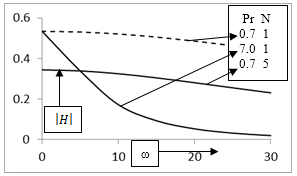Figure 7. Amplitude of Nusselt number.

## 8.png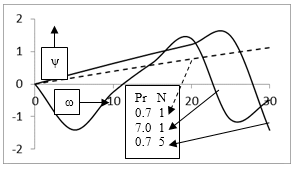Figure 8. Phase of Nusselt number.

## 9.png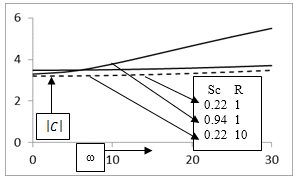Figure 9. Amplitude of Sherwood number.

## 10.png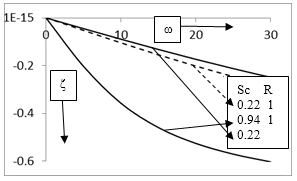Figure 10. Phase of Sherwood number.

4. Conclusions

From the discussion above following conclusions are made:

• The velocity increases with the increase of buoyancy forces due to concentration and thermal diffusions (Gr, Gm) and permeability K.

• The flow velocity decreases with the increase of M, Pr, N, Sc, R and frequency of oscillations ω.

• The skin-friction amplitude increases with the increase of Gr, K, Sc and R while decreases with the increase Gm, M, Pr and N.

• There is always a phase lead in the skin-friction.

• The phase lead of skin friction increases with the increase Gr, Gm, K and R but decreases with the increase of M, Pr, N, and Sc.

• The temperature decreases with Pr, N and ω.

• The species concentration also decreases with the increase of Sc, R and ω.

• The respective amplitudes of Nusselt number and the Sherwood number increase sharply and decrease mildly with the increase of different parameters involved.

• There is always a phase lag for the Sherwood number.

References

1. R. Muthucumarswamy and P. Ganesan, Effect of chemical reaction and injection on flow characteristics in an unsteady upward motion of an isothermal plate, J. Appl. Mech. Tech. Phys., vol. 42, No. 4, pp. 665-671, 2001.

2. R. Kandasamy K. Periasamy and K. K. Sivagnana Prabhu, Chemical reaction, heat and mass transfer on MHD flow over a vertical stretching surface with heat source and thermal stratification effects, International Journal of Heat and Mass Transfer, vol. 48, pp. 4557–4561, 2005.

3. R. Muthucumaraswamy and B. Janakiraman, Mass transfer effects on isothermal vertical oscillating plate in the presence of chemical reaction, International Journal of Applied Mathematics and Mechanics, vol. 4, no. 1, pp. 66–74, 2008.

4. U.N. Das, R. K. Deka and V. M. Soundalgekar, Effects of mass transfer on flow past an impulsively started

vertical infinite vertical plate with constant heat flux and chemical reaction, Forschung in Ingenieurwesen vol. 60, pp. 284 – 287, 1994.

5. M. A. Hossain and H. S. Takhar, Radiation effect on mixed convection along a vertical plate with uniform surface temperature, Heat and Mass Transfer, vol. 31, pp. 243–248, 1996.

6. C. Israel-Cookey , A. Ogulu and V. B. Omubo-Pepple, Influence of viscous dissipation on unsteady MHD free-convection flow past an infinite heated vertical plate in porous medium with time-dependent suction, Int. J. Heat Mass Transfer, vol. 46, pp. 2305-2311, 2003.

7. D. Ch. Kesavaiah, P. V. Satyanarayana and S. Venkataramana Effects of the chemical reaction and radiation absorption on an unsteady MHD convective heat and mass transfer flow past a semi-infinite vertical permeable moving plate embedded in a porous medium with heat source and suction, Int. J. of Appl. Math and Mech.vol. 7, pp. 52-69, 2011.

8. M. A. Hossain, M. A. Alim and D. A. S. Rees, The effect of radiation on free convection from a porous vertical plate, International Journal of Heat and Mass Transfer, vol. 42, no. 1, pp. 181–191, 1999.

9. M. A. Seddeek, Effects of radiation and variable viscosity on a MHD free convection flow past a semi-infinite flat plate with an aligned magnetic field in the case of unsteady flow, International Journal of Heat and Mass Transfer, vol. 45, no. 4, pp. 931–935, 2002.

10. R. Muthucumaraswamy and G. K. Senthil, Studied the effect of heat and mass transfer on moving vertical plate in the presence of thermal radiation, Journal of Theoretical And Applied Mechanics, vol. 31, no. 1, pp. 35–46, 2004.

11. O. Aydin and A. Kaya, Radiation effect on MHD mixed convection flow about a permeable vertical plate, Heat and Mass Transfer, vol. 45, no. 2, pp. 239–246, 2008.

12. D. S. Chauhan and P. Rastogi, Radiation effects on natural convection MHD flow in a rotating vertical porous channel partially filled with a porous medium, Applied Mathematical Sciences, vol. 4, no. 13–16, pp. 643–655, 2010.

13. S. Y. Ibrahim and O. D. Makinde, Radiation effect on chemically reacting magnetohydrodynamics (MHD) boundary layer flow of heat and mass transfer through a porous vertical flat plate, International Journal of Physical Sciences, vol. 6, pp. 1508–1516, 2011.

14. M. Sudheer Babu and P. V. Satya Narayan, Effects of the chemical reaction and radiation absorption on free convection flow through porous medium with variable suction in the presence of uniform magnetic field, Journal of Heat and Mass Transfer, vol. 3, pp. 219–234, 2009.

15. D. Pal and B. Talukdar, Combined effects of Joule heating and chemical reaction on unsteady magnetohydrodynamic mixed convection of a viscous dissipating fluid over a vertical plate in porous media with thermal radiation, Mathematical and Computer Modelling, vol. 54, pp. 3016–3036, 2011.

16. K. D. Alagoa, G. Tay and T. M. Abbey, Radiative and free convective effects of a MHD flow through a porous medium between infinite parallel plates with time-dependent suction. Astrophysics and Space Science, vol. 260, pp. 455-468, 1999.

17. K. D. Singh, Unsteady free convection flow past a hot vertical porous plate with variable temperature, Proc. Indian Natn. Sci. Acad., vol. 58, pp. 537-544, 1992.

18. K. D. Singh and Khem chand, Unsteady free convective MHD flow past a vertical porous plate with variable temperature, Proc. Nat. Acad. Sci. India, vol. 70, pp. 49-58, 2000.

19. K. Sumathi, S. Anuradha and T. Arunachalam, Heat and mass transfer in an unsteady three dimensional mixed convection flow past an infinite vertical porous

plate with cosinusoidally fluctuating temperature, International J. Engineering Science and Technology, vol. 3, pp. 8569-8578, 2011.

20. R. Kumar and K. D. Singh, Unsteady MHD flow of radiating and reacting fluid past a vertical porous plate with cosinusoidally fluctuating temperature, Int. J. of Appl. Math. and Mech., vol. 7, pp.19-33, 2011.

21. R. Siegel and J. R. Howell, Thermal Radiation Heat Transfer, New York: Hemisphere, USA, 1972.